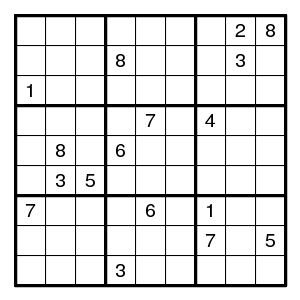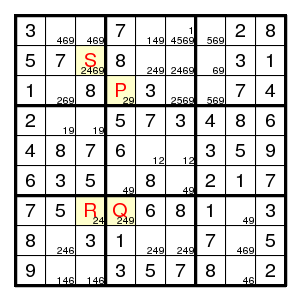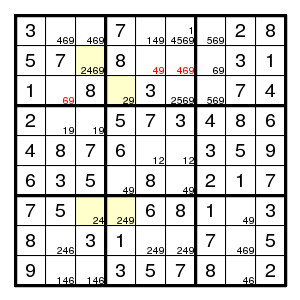Prev Up Next

# Alternating pairs

Call two positions on a sudoku grid adjacent when they are in the same row or column or box. Then two adjacent positions have different values. Recall that we used 'pair' (for some digit d) for two adjacent positions one of which must contain d.

Suppose we have a chain A=B-C=D (or A=B-C=D-E=F etc.), where every odd link is a pair (for the same digit d) and the remaining links are ordinary adjacencies. Then a common neighbour of the two ends of the chain is not d.

(Indeed, if for example the chain is A=B-C=D-E=F and X is adjacent to both A and F so that we have X-A=B-C=D-E=F-X, and X is d, then A is not, so B is, so C is not, ..., so F is, so X is not.)

Terminology The forcing chains described above are called 'x-cycles'.

Look at an example.The starting configuration leads without problems to the situation on the right. It has the chain P=Q-R=S for the digit 2 indicated in yellow. Therefore the three common neighbours of P and S do not have a 2. We obtainand the rest is straightforward.

(There was one more application in the same diagram, also for the digit 2, namely (8,2)=(3,2)-(3,4)=(7,4) where the three positions (7,3), (8,5), (8,6) are common neighbours of the endpoints, and consequently cannot be 2.)

Terminology This argument (for a chain of length 3) is also known as 'Turbot Fish'.